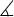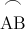Sunday , 25 September 2022

# Geometry Symbols

Geometry Symbols – List of all geometry symbols and signs – meaning and examples.

Symbol Symbol Name Meaning / definition Example
angle formed by two rays ∠ABC = 30°measured angleABC = 30°spherical angleAOB = 30°
right angle = 90° α = 90°
º degree 1 turn = 360° α = 60°
´ arcminute 1º = 60´ α = 60°59′
´´ arcsecond 1´ = 60´´ α = 60°59’59”line infinite line
AB line segment line from point A to point Bray line that start from point Aarc arc from point A to point B= 60°
| perpendicular perpendicular lines (90° angle) AC | BC
|| parallel parallel lines AB || CD
congruent to equivalence of geometric shapes and size ∆ABC ≅ ∆XYZ
~ similarity same shapes, not same size ∆ABC ~ ∆XYZ
Δ triangle triangle shape ΔABC ≅ ΔBCD
|xy| distance distance between points x and y | xy | = 5
π pi constant π = 3.141592654…
is the ratio between the circumference and diameter of a circle
c = π·d = 2·π·r